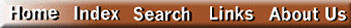News    Archives    Mapby Miguel A Sepulveda About the author: Graduated from the University of Washington (USA) in 1993. Work in scientific research in Spain, Israel, Japan and the US. Discovered Linux for the first time around kernel 0.98 (Love at first sight). Now is Editor-in-Chief of the LinuxFocus magazine in his free time. Content:

## OpenGL Programming: Simple Polygon RenderingAbstract:

This article describes the first steps into any OpenGL application and tells us how to render simple polygons in 2D.

_________________ _________________ _________________

### Introduction

This is the first article in a series on OpenGL, an industry standard for 2D/3D graphics (see also What is OpenGL). We will assume that the reader is familiar with his/hers C development platform, and has some knowledge of the GLUT library (otherwise just follow the "GLUT programming" series of articles in this magazine). Under Linux we recommend the usage of the Mesa-library which is a wonderful freeware implementation of OpenGL. Now there is even hardware support for Mesa (see 3Dfx graphics card).

Every presentation of new OpenGL commands will be accompanied by an example that tries to exploit its functionality, at least we will try !. By the end of our series we will present you with the source code of a game simulation completely written in OpenGL.

Before getting started I would like to mention that since I am a scientist, most of my experience with OpenGL is as a tool to write simulations of real quantum and classical systems. So my examples are a bit bias ;-). I hope readers find these examples accessible or at least amusing. If you would like to see other kinds of examples just let me know.

OpenGL is often associated with 3D graphics, fancy special effects, complex models with realistic light modeling etc.. However it is also a 2D graphics rendering machine. This is important because there are many things you can learn to do in 2D before starting to learn about the complexities of 3D perspectives, model rendering, lights, camera position, etc.. A large number of engineering and science applications can be render in 2D. So let us first learn how to do some simple 2D animations.

### Drawing Points

OpenGL has only a few geometric primitives: points, lines, polygons. All of them are described in terms of their respective vertices. A vertex is characterized by 2 or 3 floating points, the Cartesian coordinates of the vertex, (x,y) in 2D and (x, y, z) in 3D. While Cartesian coordinates are the most common, in computer graphics there is also the homogeneous coordinate system in which every point is described by 4 floating points (x, y, z, w). We will come back to them after covering some elementary notions of 3D rendering.

Since in OpenGL all geometric objects are eventually described as an ordered set of vertices, there is a family of routines to declare a vertex. Its syntax is:

void glVertex{234}{sifd}[v](TYPE coords);

Get familiar with this notation. The curly brackets indicate part of the name of the routine. The routines can take 2, 3 or 4 parameters in either short, long, float or double type. Optionally these parameters can be supplied in a vector form, in which case we would use the v-type routines. Here are some examples:

void glVertex2s(1, 3);
void glVertex2i(23L, 43L);
void glVertex3f(1.0F, 1.0F, 5.87220972F);

float vector;
void glVertex3fv(vector);

To simplify all these routines are refered to as glVertex*.

OpenGL interprets any sequence of vertices according to its context. The context is declared by the pair of routines glBegin(GLenum mode) and glEnd(), any glVertex* statements executed between the two are interpreted according to the value of mode, for example:
glBegin(GL_POINTS);
glVertex2f(0.0, 0.0);
glVertex2f(1.0, 0.0);
glVertex2f(0.0, 1.0);
glVertex2f(1.0, 1.0);
glVertex2f(0.5, 0.5);
glEnd();

draws 5 points in 2D with the coordinates specified. GL_POINTS is one of the labels defined in the OpenGL header file <GL/gl.h>. There are many other modes available but we will review then as necessary.

Every point is drawn with the color currently stored in the OpenGL state variable associated with the color buffer. To change the current color, use the family of routines glColor*; there is a lot to say about selecting and manipulating colors (there will be another article only on this subject). For the moment, we will be using three floating point numbers from 0.0 to 1.0 - this is the RGB (Red-Green-Blue) encoding;
glColor3f(1.0, 1.0, 1.0); /* White */
glColor3f(1.0, 0.0, 0.0); /* Red */
glColor3f(1.0, 1.0, 0.0); /* Magenta */
etc...

This is already enough material to write our first two examples of code. The first example is a simple OpenGL program that draws a number of orbits in a chaotic map (The standard map). If the reader is not familiar with mappings and the standard map in particular, it does not matter. To put it simply, a map takes a point and generates a new one using a well defined formula:

yn+1 = yn + K sin(xn)
xn+1 = xn + yn+1

In the case of the standard map it represents a model for the trace left by a charged particle that circles around the tori of an accelerator of particles and crosses a section plane of the accelerator. Studying the properties of this and other maps is important in physics because it helps us understand the stability of the charged particle confined in the cyclotron. The standard map is very cool because for some values of its parameter, K clearly shows a mixture of chaotic and trapped motion. Finally even those who are not really interested in physics but still want to develop some nice graphics code should pay some attention to maps and their properties, many of the algorithms for generating textures, fire flares, trees, terrain, etc.. are based on fractal maps.

Here is the code example1.c:

```
#include <GL/glut.h>
#include <math.h>

const  double pi2 = 6.28318530718;

void NonlinearMap(double *x, double *y){
static double K = 1.04295;
*y += K * sin(*x);
*x += *y;
*x = fmod(*x, pi2);
if (*x < 0.0) *x += pi2;
};

void winInit(){
/* Set system of coordinates */
gluOrtho2D(0.0, pi2, 0.0, pi2);
};

void display(void){
const int    NumberSteps = 1000;
const int    NumberOrbits = 100;
const double Delta_x = pi2/(NumberOrbits-1);
int step, orbit;

glColor3f(0.0, 0.0, 0.0);
glClear(GL_COLOR_BUFFER_BIT);
glColor3f(1.0, 1.0, 1.0);

for (orbit = 0; orbit < NumberOrbits; orbit++){
double x, y;
y = 3.1415;
x = Delta_x * orbit;

glBegin(GL_POINTS);
for (step = 0; step < NumberSteps; step++){
NonlinearMap(&x, &y);
glVertex2f(x, y);
};

glEnd();
};

for (orbit = 0; orbit < NumberOrbits; orbit++){
double x, y;
x = 3.1415;
y = Delta_x * orbit;

glBegin(GL_POINTS);
for (step = 0; step < NumberSteps; step++){
NonlinearMap(&x, &y);
glVertex2f(x, y);
};
glEnd();

};
};

int main(int argc, char **argv) {
glutInit(&argc, argv);
glutInitDisplayMode(GLUT_SINGLE | GLUT_RGBA);
glutInitWindowPosition(5,5);
glutInitWindowSize(300,300);
glutCreateWindow("Standard Map");

winInit();
glutDisplayFunc(display);
glutMainLoop();

return 0;
}

```
Please read the Programming GLUT article to understand the glut* routines, most of this code came from there. The graphics window is opened in single buffer and RGB mode. Then a callback function named display() draws the map: first we select the color black for the background; glClear(GL_COLOR_BUFFER_BIT) resets the color buffer to the current color (black), next after selecting white color with glColor, we run the NonlinearMap() a number of times and plot the points with glVertex* in GL_POINTS mode. Really simple.

Notice that in the window initialization routine winInit() there is a single statement from the OpenGL Utility toolkit, gluOrtho2D(). This routine sets a 2D orthogonal system of coordinates. The parameters passed are "minimum x, maximum x, minimum y, maximum y".
I have chosen a single mode window and a large number of points so that you have a chance to see the image as it is being drawn. This is common of single mode with large and time consuming images, things appear on your screen as they are invoked with OpenGL routines.

After running ../../common/January1998/example1 you should see this image: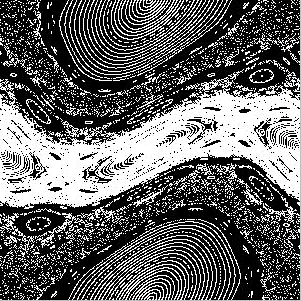Let's go now to the second program, example2.c:

```
#include <GL/glut.h>
#include <math.h>

const  double  pi2 = 6.28318530718;
const  double  K_max = 3.5;
const  double  K_min = 0.1;
static double  Delta_K = 0.01;
static double  K = 0.1;

void NonlinearMap(double *x, double *y){
/* Standard Map */
*y += K * sin(*x);
*x += *y;

/* Angle x is module 2Pi */
*x = fmod(*x, pi2);
if (*x < 0.0) *x += pi2;
};

/* Callback function:
What to do in absence of use input */
void  idle(void){
/* Increase the stochastic parameter */
K += Delta_K;
if(K > K_max) K = K_min;

/* Redraw the display */
glutPostRedisplay();
};

/* Initialization for the graphics window */
void winInit(void){
gluOrtho2D(0.0, pi2, 0.0, pi2);
};

/* Callback function:
What to do when the display needs redrawing */
void display(void){
const int    NumberSteps = 1000;
const int    NumberOrbits = 50;
const double Delta_x = pi2/(NumberOrbits-1);
int step, orbit;

glColor3f(0.0, 0.0, 0.0);
glClear(GL_COLOR_BUFFER_BIT);
glColor3f(1.0, 1.0, 1.0);

for (orbit = 0; orbit < NumberOrbits; orbit++){
double x, y;
y = 3.1415;
x = Delta_x * orbit;

glBegin(GL_POINTS);
for (step = 0; step < NumberSteps; step++){
NonlinearMap(&x, &y);
glVertex2f(x, y);
};
glEnd();
};

for (orbit = 0; orbit < NumberOrbits; orbit++){
double x, y;
x = 3.1415;
y = Delta_x * orbit;

glBegin(GL_POINTS);
for (step = 0; step < NumberSteps; step++){
NonlinearMap(&x, &y);
glVertex2f(x, y);
};
glEnd();
};

glutSwapBuffers();
};

int main(int argc, char **argv)  {
/* GLUT Initializations */
glutInit(&argc, argv);
glutInitDisplayMode(GLUT_DOUBLE | GLUT_RGBA);
glutInitWindowPosition(5,5);
glutInitWindowSize(300,300);

/* Open Window */
glutCreateWindow("Order to Chaos");

/* Window initializations */
winInit();

/* Register callback functions */
glutDisplayFunc(display);
glutIdleFunc(idle);

/* Launch event processing */
glutMainLoop();

return 0;
}

```
This program is based on ../../common/January1998/../../common/January1998/example1.c, the main difference is that the window is opened in a double buffer mode, and the map parameter K is a variable that changes during the life of the program. There is a new callback function idle() registered to the GLUT event processor by glutIdleFunc(). This function has a special meaning; it gets run every so often by the event processor in the absence of user input. The idle() callback function is ideal for programming animations. In ../../common/January1998/example2, it serves the purpose of changing slightly the value of the map parameter. At the end of idle() there is another useful GLUT statement, glutPostResDisplay() which redraws the window preserving the previous window's initializations. In general, it is more efficient than simply calling display() again.

Another difference worth noticing is the use of glutSwapBuffers() at the end of display(). The window was initialized in double buffer mode, therefore all the rendering directives are applied to the hidden buffer; the user cannot see the image being draw in this case. After the whole image (frame) has been finished then it is made visible by switching hidden and visible buffers with glutSwapBuffers(). Without this technique the animation will not run smoothly.

Here are some of the frames displayed during the animation: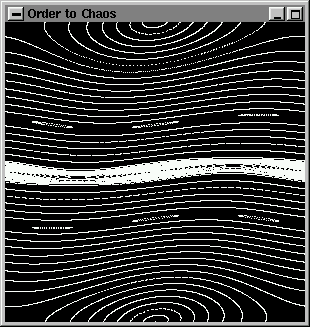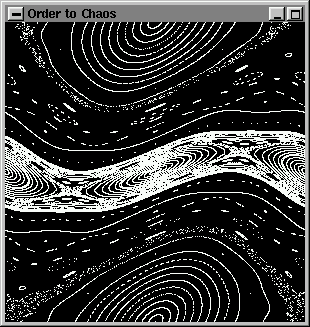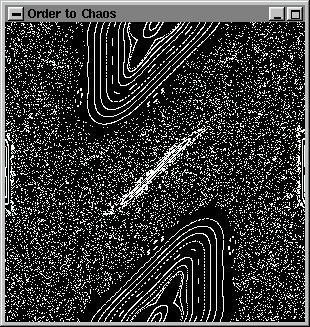IMPORTANT: The display() callback function always gets invoked at least once, before idle(). Keep this in mind when writing your animations and deciding what goes to display() and what to idle().

### Drawing Lines and Polygons

example3.c

As previously mentioned glBegin(GLenum mode) accepts various modes and the sequence of vertices v0, v1,v2, v3,v4,... vn-1 declared afterwards are interpreted accordingly. The possible values for mode and the actions taken are:

• GL_POINTS    Draws a points at each of the n vertices.
• GL_LINES    Draws a series of unconnected lines. The segments are drawn between v0 and v1, v2 and v3,...etc. If n is odd vn-1 is ignored.
• GL_POLYGON    Draws a polygon using v0, v1,..,vn-1 as vertices. n must be at least 3 or nothing is drawn, also the polygon can not intersect itself and must be convex (due to the hardware's algorithm limitations).
• GL_TRIANGLES    Draws a series of triangles using vertices v0, v1 and v2, then v3, v4 and v5 etc. If n is not a multiple of 3 the remaining points are ignored.
• GL_LINE_STRIP    Draws a line from v0 to v1, them from v1 to v2 and so on. Finally from vn-2 to vn-1 for a total of n-1 line segments. There are no restrictions on the vertices describing a line strip, lines can intersect arbitrarily.
• GL_LINE_LOOP    Same as GL_LINE_STRIP except that a final line segment is drawn from vn-1 to v0, closing the loop.
• GL_QUADS    Draws a series of quadrilaterals using vertices v0, v1, v2, v3 and v4, v5, v6, v7 and so on.
• GL_QUAD_STRIP    Draws a series of quadrilaterals using vertices v0, v1, v3, v2 then v2, v3, v5, v4 and so on.
• GL_TRIANGLE_STRIP    Draws a series of triangles using vertices in the following order v0, v1, v2, then v2, v1, v3, then v2, v3, v4, etc. The ordering is to ensure that the triangles has the correct orientation and the strip can be used to form part of a surface.
• GL_TRIANGLE_FAN    Similar to GL_TRIANGLE_STRIP except that the triangles are v0, v1, v2, then v0, v2, v3, then v0, v3, v4, and so on. All the triangles have v0 as a common vertix.
In our third example, another animation, we make use of GL_LINES and GL_POLYGON. Compile the program, then take a look at the source code and see how it works. It is basically very similar to ../../common/January1998/../../common/January1998/example2.c, now the image drawn is a very simple pendulum. The animation simulates the motion of an ideal pendulum. Here is a snapshot of the animation: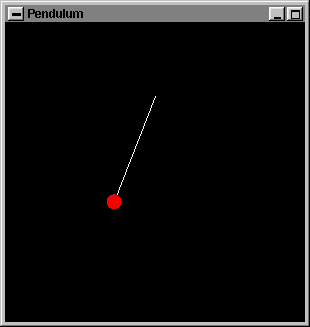As before there is an idle() callback function whose aim here is to keep the clock running (updating the variable time). The display() draws two objects; the pendulum cord and weight (in white and red respectively). The motion of the pendulum coordinates is implicit in the formulas for xcenter and ycenter:

```

void display(void){
const double delta_theta = pi2/20;
double xcenter , ycenter;
double x, y;
double theta = 0.0;

double current_angle = cos(omega * time);

glColor3f(0.0, 0.0, 0.0);
glClear(GL_COLOR_BUFFER_BIT);
glColor3f(1.0, 1.0, 1.0);

/* Draw pendulum cord */
glColor3f(1.0, 1.0, 1.0);
glBegin(GL_LINES);
glVertex2f(0.0, 0.0);
xcenter = -cord_length * sin(current_angle);
ycenter = -cord_length * cos(current_angle);
glVertex2f(xcenter, ycenter);
glEnd();

/* Draw pendulum dish */
glColor3f(1.0, 0.0, 0.0);
glBegin(GL_POLYGON);
while (theta <= pi2) {
x = xcenter + radius * sin(theta);
y = ycenter + radius * cos(theta);
glVertex2f(x, y);
theta += delta_theta;
};
glEnd();

glutSwapBuffers();
};

```

### Exercises

Here are some suggestions for practicing what you have learned so far:

• In example1.c try other maps. Go to the library and pick any book on Chaos and Fractals, surely you will find many examples. Experiment changing parameters, system of coordinates, and applying several maps consecutively before drawing the points. Have fun with it.
• In example2.c you could add colors to each of the points. For example, a very interesting color coding would be based on assigning to each dot a color according to the local stability of the orbit (Physics Review Letters Vol 63, (1989) 1226), when the trajectory goes through a chaotic region it becomes more red. For instance, while near stable islands it could become more blue. If you code this effect, the fractal nature of our example map will become obvious. It is a bit advanced for those of you without course work on differential equations, but it is worthwhile learning about it if you want to take advantage of mappings and fractals in your computer graphics.
• In example3.c , try changing the type of lines used for drawing the disc. Use GL_LINES, GL_TRIANGLES, etc.. See what happens. Try optimizing the disc generation, it is not necessary to evaluate sines and cosines so many times for drawing the same disc in each frame, you could save it into an array. Using polygon drawing try attaching boxes, diamonds, or whatever to the end of the pendulum. Write two pendulums per frame, moving independently or even colliding with each other.

### Next Time

This is all for now. There are still many things to discuss about polygons. In the next issue (March 1998) we will continue to explore polygons, modeling and cover in more detail some of the commands your are already familiar with.